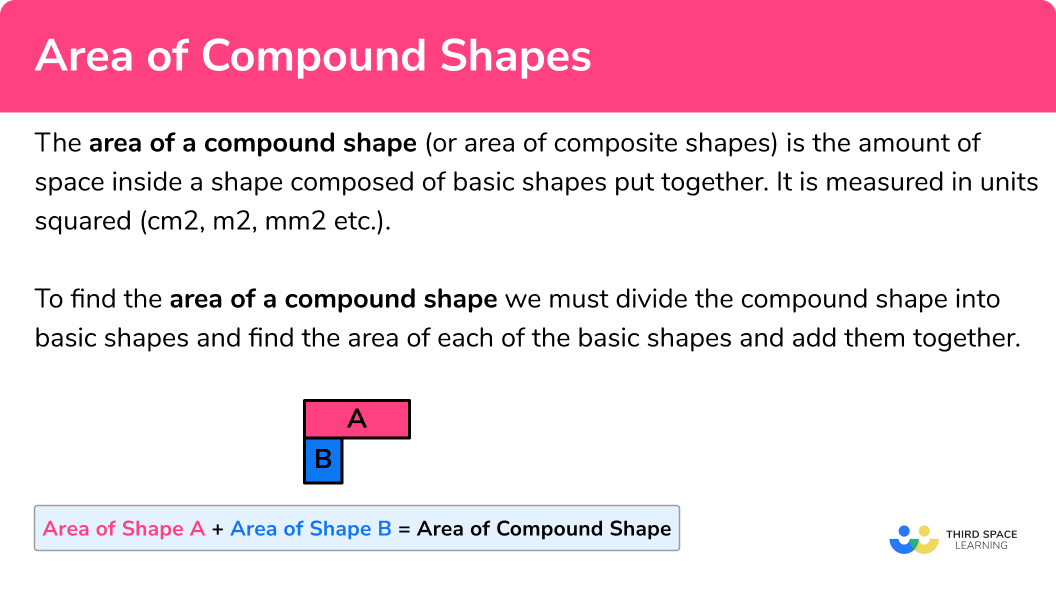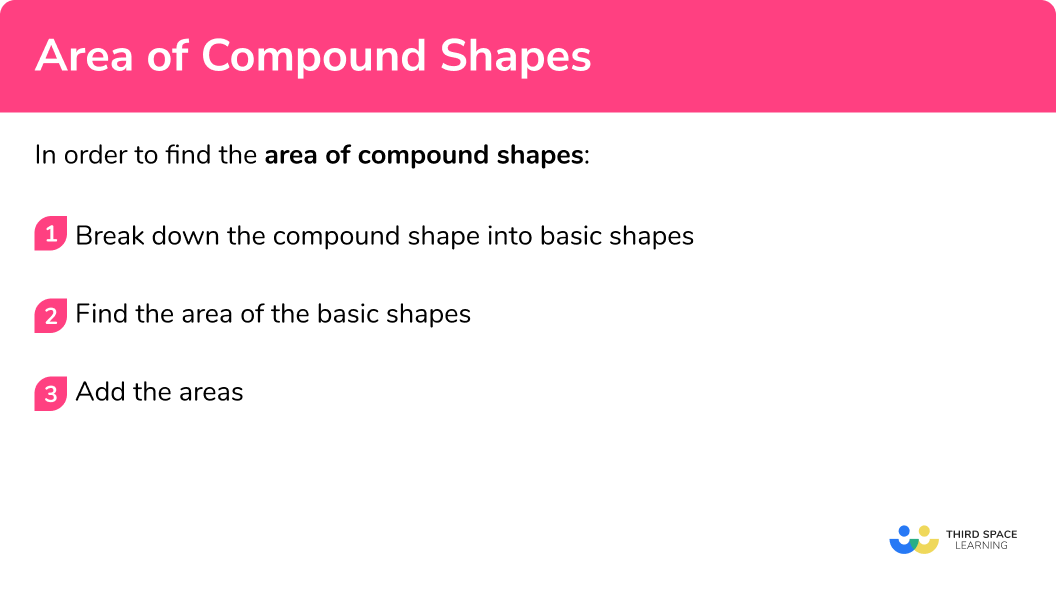GCSE Maths Geometry and Measure Area

Area Of Compound Shapes

# Area Of Compound Shapes

Here we will learn about the area of compound shapes, including questions involving rectilinear shapes, composite shapes, missing side lengths and unit conversion.

There are also area of compound shape worksheets based on Edexcel, AQA and OCR exam questions, along with further guidance on where to go next if you’re still stuck.

## What is the area of compound shapes?

The area of compound shapes (also known as the area of composite shapes) is the amount of space inside a shape composed of basic shapes put together. It is measured in units squared ( cm^2 , m^2, mm^2 etc.).

Compound shapes can also be called composite shapes.

To find the area of compound shapes we must divide the compound shape into basic shapes and find the area of each of the basic shapes and add them together.

Our final answer must be in square units e.g. square centimetres (cm^2) , square meters (m^2) , square feet (ft^2), etc.

### What is the area of compound shapes?## How to find the area of compound shapes

In order to find the area of compound shapes:

1. Break down the compound shape into basic shapes.
2. Find the area of the basic shapes.

### Explain how to find the area of compound shapes## Related lessons on area

Area of compound shapes is part of our series of lessons to support revision on area. You may find it helpful to start with the main area lesson for a summary of what to expect, or use the step by step guides below for further detail on individual topics. Other lessons in this series include:

## Area of compound shapes examples

### Example 1: all side lengths given (area of the L shape)

Find the area of the compound shape below:

1. Break down the compound shape into basic shapes.

Split the compound shape into two rectangles. We can do this in two ways:For the purposes of this question we will use the first way.

2Find the area of the basic shapes.

Let us label the two rectangles A and B :

Area of Rectangle A :

\begin{aligned} \text { Area }_{A} &=\text { length } \times \text { width } \\\\ \text { Area }_{A} &=6 \times 5 \\\\ &=30 \end{aligned}

Area of Rectangle B :

\begin{aligned} \text { Area }_{B} &=\text { length } \times \text { width } \\\\ \text { Area }_{B} &=9 \times 4 \\\\ &=36 \end{aligned}

\begin{aligned} \text { Compound Area } &=\text { Area of } A+\text { Area of } B \\\\ &= 30+36 \\\\ &=66 \end{aligned}

\text { Area }=66 \mathrm{~m}^{2}

### Example 2: missing lengths

Find the area of the compound shape below:

Break down the compound shape into basic shapes.

Find the area of the basic shapes.

### Example 3: worded question

The wall shown below needs to be painted. Each tin of paint covers a distance of 4 square metres. How many tins of paint will be needed to cover the entire wall?

Break down the compound shape into basic shapes.

Find the area of the basic shapes.

### Example 4: compound shapes involving non-rectangular shapes

Find the area of the compound shape below:

Break down the compound shape into basic shapes.

Find the area of the basic shapes.

### Example 5: missing side lengths

Calculate the value of x in the compound shape below:

Break down the compound shape into basic shapes.

Find the area of the basic shapes.

### Example 6: worded question

Below is a blueprint for a garden to be created in the middle of town. It will feature a triangular flowerbed and the remainder of the garden will be covered in grass.

Each roll of grass costs £24 and covers a distance of 3 square metres. How much will it cost to cover the garden with grass?

Break down the compound shape into basic shapes.

Find the area of the basic shapes.

### Common misconceptions

• Using incorrect units for the answer

A common error is to forget to include squared units when asked to calculate area.

Forgetting to convert measures to a common unit

Before using the formula for calculating the area of a rectangle, pupils must look at the units given in the question. If different units are given e.g. length = 4m and width = 3cm pupils must convert them either both to cm or both to m.

• Composite shapes

A composite shapes is the same as a compound shape

### Practice area of compound shapes questions

1. Find the area of the compound shape below:80m^265m^250m^280mSplit into two rectangles:Area of A : 10 \times 5=50

Area of B : 4 \times 4=16

Compound Area: 50+16=66m^2

2. Find the area of the compound shape below:50m^225m^2104m^2110m^2Split into two rectangles:Area of  A : 10 \times 5=50

Area of  B : 9 \times 6=54

Compound Area: 50+16=104m^2

3. The wall shown below needs to be painted. Each tin of paint covers a distance of 4 square metres. How many tins of paint will be needed to cover the entire wall?4216641150Split into three rectangles:Area of A : 15 \times 5=75

Area of B : 8 \times 2=16

Area of C : 15 \times 5=75

Compound Area: 75+16+75=166m^2

Then divide by 4 to get the number of tins.

4. Find the area of the compound shape below:95,000cm^264,600cm^2710cm^279,800cm^2Split into one rectangle and two triangles:Area of A : \frac{1}{2}(190\times80)=7600

Area of B : \frac{1}{2}(190\times80)=7600

Area of C : 170 \times 380=64600

Compound Area: 7600+7600+646000=79,800m^2

5. Calculate the value of x in the compound shape below:4mm7mm77mm^222mmSplit into two rectangles:Area of B : 4\times 11=44

\begin{aligned} &56-44=12\\ &12\div3=4\\ &11-4=7mm \end{aligned}

6. An architect is developing a blueprint for one floor of a house which is shown below. The shaded region represents the floor and will need to be fully tiled. Each square tile has a width of 12cm . How many tiles will be needed to cover the entire floor?717749316Area of A : 7 \times 11=77

Area of B : \frac{1}{2}(4\times3)=6

\begin{aligned} &77-6=71m^2 \\ &12cm = 0.12m \end{aligned}

Area of 1 tile: = 0.12 \times 0.12=0.0144m^2

\begin{aligned} &71\div0.0144=4930.56\\ \end{aligned}

4931 tiles

### Area of compound shapes GCSE questions

1. The diagram below shows a shape. Work out the area of the shape.(3 marks)

6 \times 7=42 seen

(1)

15\times5=75 seen or 5m seen as the missing vertical length

(1)

117m^2 (cao including units)

(1)

2. (a) A farmer owns the cattle field shown below. Each cattle costs approximately £450 and the farmer estimates that each cow requires a minimum of 4 square metres to graze freely.

What is the maximum number of cattle that could fit on the field?(b)      How much would it cost to acquire all the cattle?

(7 marks)

(a)

12 \times 5=60 seen (area of the rectangle)

(1)

\frac{1}{2}(12\times3)=18 seen (area of the triangle)

(1)

60+18=78 seen (total area)

(1)

78\div4=19.5

(1)

19 (cao)

(1)

(b)

19\times450

(1)

£8550

(1)

3. What is the perimeter of the shape below?(6 marks)Splits into two rectangles and calculates \times by using vertical side lengths given (20-7) or 13 seen

(1)

Calculates area of A by multiplying 13 \times 8 or 104 seen

(1)

132-104 = 28 seen B = 28cm^2

(1)

z=28\div7, \; z = 4

(1)

Using horizontal lengths to calculate y, \; y=8-4, \; y=4

(1)

P=8+20+4+13+4+7 or 56cm seen

(1)

## Learning checklist

You have now learned how to:

• Calculate and solve problems involving composite shapes
• Calculate and solve problems involving the perimeter and area of triangles, parallelograms and trapezia

## Still stuck?

Prepare your KS4 students for maths GCSEs success with Third Space Learning. Weekly online one to one GCSE maths revision lessons delivered by expert maths tutors.

Find out more about our GCSE maths tuition programme.